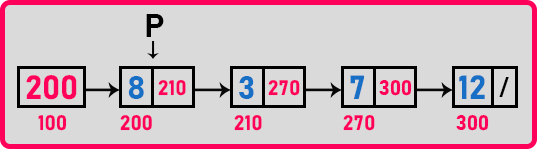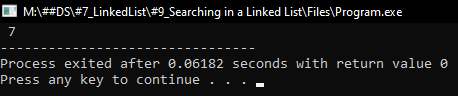# Linear Search in Linked List

## Linear Search in Linked List using C Language with Examples:

In this article, I am going to discuss Linear Search in a Linked List using C Language with Examples. Please read our previous article, where we discussed Finding Maximum Element in a Linked List using C Language with Examples.

We know about two search procedures that are linear search and binary search. Linear Search is sequential as it will check for the elements one by one and Binary Search will work only on the target list and it will check in the middle of the list. If the element is found it’s ok, if it is small, it will check on the left side otherwise it will check on the right side.

We know that we cannot perform the binary search upon a linked list because we cannot directly jump in the middle of a linked list. We have to traverse it from starting. So, traversing takes the order of n time, we cannot reach in the middle in constant time. So binary search is not suitable. So, we look at only linear search.

##### How to Perform Linear Search in a Linked List

Let us understand linear search.Suppose we are searching for a key ‘12’. We will start looking for the key from the first node.

1. First, ‘p’ will point to the address ‘200’ and its data is ‘8’. So, this is not the key.
2. Then ‘p’ will point to the address ‘210’ and its data is ‘3’. So, this is also not the key.
3. Then ‘p’ will point to the address ‘270’ and its data is ‘7’. This is also not the key.
4. Then ‘p’ will point to the address ‘300’ and its data is ‘12’. This is the key we are searching for.

So, it means we have to traverse the linked list and go on looking for the key element. It is the same as traversing but the thing is we have to stop when we found the key. Now let us write a function for searching.

##### Function to Search a Particular Element in a Linked List using C Language:
###### Iterative Function:
```Node* LSearch (Node * p, int key)
{
while (p != NULL)
{
if (key == p->data)
return p;
p = p->next;
}
return NULL;
}
```
###### Recursive Function:
```Node* RSearch (Node * p, int key)
{
if (p == NULL)
return NULL;
if (key == p->data)
return p;
return RSearch (p->next, key);
}
```

Now let us write the full program.

##### Program to Search a Particular Element in a Linked List using Linear Search in C Language:
```#include <stdio.h>
#include <stdlib.h>
struct Node
{
int data;
struct Node *next;
}   *first = NULL;

void Create(int A[], int n)
{
int i;
struct Node *t, *last;
first = (struct Node *) malloc(sizeof (struct Node));
first->data = A;
first->next = NULL;
last = first;

for (i = 1; i < n; i++)
{
t = (struct Node *) malloc(sizeof (struct Node));
t->data = A[i];
t->next = NULL;
last->next = t;
last = t;
}
}

struct Node* LSearch(struct Node *p, int key)
{
while (p != NULL)
{
if (key == p->data)
return p;
p = p->next;
}
return NULL;
}

struct Node* RSearch(struct Node *p, int key)
{
if (p == NULL)
return NULL;
if (key == p->data)
return p;
return RSearch (p->next, key);
}

int main()
{
struct Node *temp;
int A[] = { 8, 3, 7, 12 };
Create(A, 4);
temp = LSearch(first, 7);
printf (" %d", temp->data);
return 0;
}
```
###### Output:In the next article, I am going to discuss Improve Searching in a linked list using C Language with Examples. Here, in this article, I try to explain How to Implement Linear Search in a Linked List using C Language with Examples and I hope you enjoy this Searching an Element using Linear Search in a Linked List using C Language with Examples article.